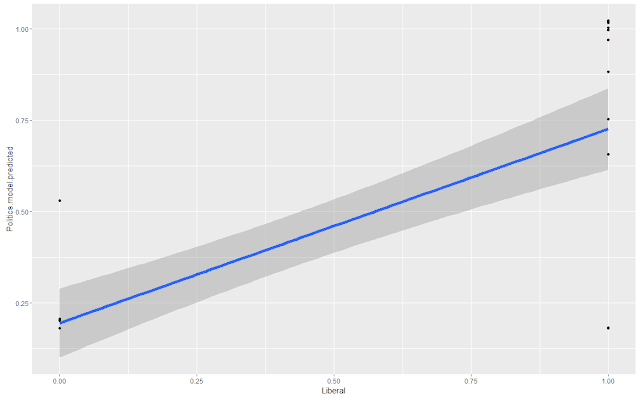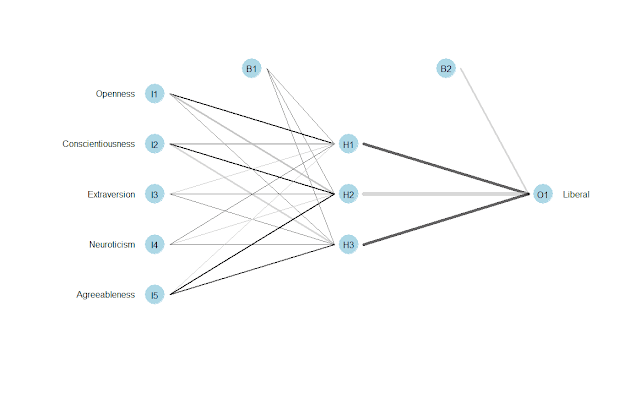### Neural Networks in R (Part 2 of 4) - caret and nnet on State 'Personality' and Political Outcomes

This is a continuation of a prior post, Neural Networks (Part 1 of 4) - Logistic Regression and neuralnet on State 'Personality' and Political Outcomes, the second in a series exploring neural networks.

This post is a demonstration using the caret and nnet packages on aggregate Big Five traits per state and political leanings. Given the small sample size, there was a lower predictive ability using the train/test scenario using 80%, around .66, versus the entire set, which was correct at .83. This is evident in the graphs using lm() and stat_smoot().

The code is below, as are some related graphs. Source data is here.

``````
################################################
# Neural Net with nnet and caret
################################################

library(nnet)
library(caret)

# train
Politics.model <- train(equation, Politics.df, method = 'nnet', linout = TRUE, trace = FALSE)
Politics.model.predicted <- predict(Politics.model, Politics.df)

# classification result
#plot(Politics.model.predicted)

Politics.model.combined <- cbind(Politics.model.predicted, Politics.df)
#View(Politics.model.combined)

# percent correct using nnet & caret
Politics.model.combined\$predictionBinary <- ifelse(Politics.model.combined\$Politics.model.predicted > .5, 1, 0)
Politics.model.combined\$Correct <- ifelse(Politics.model.combined\$predictionBinary == Politics.model.combined\$Liberal, 1, 0)
(Correct.nnet <- paste("caret using nnet - Correct (%) = ", (sum(Politics.model.combined\$Correct) / length(Politics.model.combined\$Correct))))

# display neural net model
# install.packages('NeuraNetTools')
library(NeuralNetTools)
plotnet(Politics.model, alpha = 0.6)# graph log regression
library(ggplot2)
plotPart1 <- ggplot(data = Politics.model.combined, aes(y = Politics.model.predicted, x = Liberal))
plotPart1 + geom_point() + stat_smooth(method = "lm", formula = y ~ x, size = 2)################################################
# Neural Net with nnet and caret
# Train / Test scenario
################################################

# traing and testing a model
Politics.training <- createDataPartition(y = Politics.df\$Liberal, p = 0.8, list = FALSE)

train.set <- Politics.df[Politics.training,]
test.set <- Politics.df[ - Politics.training,]
nrow(train.set) / nrow(test.set) # should be around 4

Politics.training.model <- train(equation, train.set, method = "nnet", trace = FALSE)
Politics.training.prediction <- predict(Politics.training.model, test.set)

Politics.training.combined <- as.data.frame(cbind(Politics.training.prediction, test.set))
# View(Politics.training.combined)

# percent correct for test set
# percent correct using nnet & caret
Politics.training.combined\$predictionBinary <- ifelse(Politics.training.combined\$Politics.training.prediction > .5, 1, 0)
Politics.training.combined\$Correct <- ifelse(Politics.training.combined\$predictionBinary == Politics.training.combined\$Liberal, 1, 0)
(Correct.test <- paste("caret using nnet (Test/Train) - Correct (%) = ", (sum(Politics.training.combined\$Correct) / length(Politics.training.combined\$Correct))))

# display neural net model
# install.packages('NeuraNetTools')
library(NeuralNetTools)
plotnet(Politics.training.model, alpha = 0.6)# graph result, prediction versus actual, and add linear model line
library(ggplot2)
plotPart1 <- ggplot(data = Politics.training.combined, aes(y = Politics.training.prediction, x = Liberal))
plotPart1 + geom_point() + stat_smooth(method = "lm", formula = y ~ x, size = 2)``````